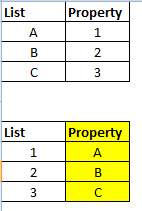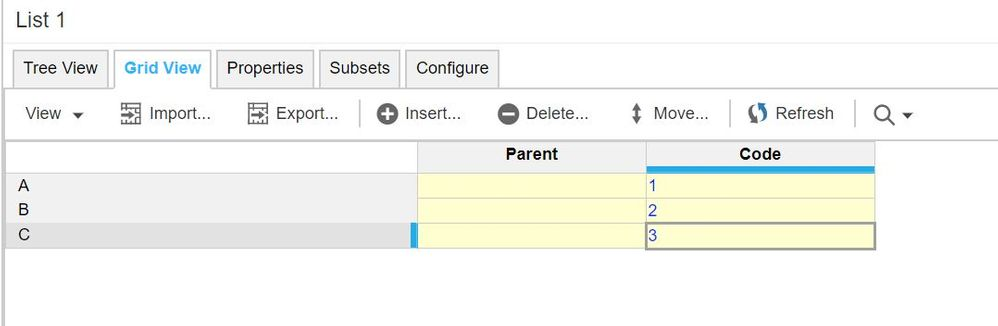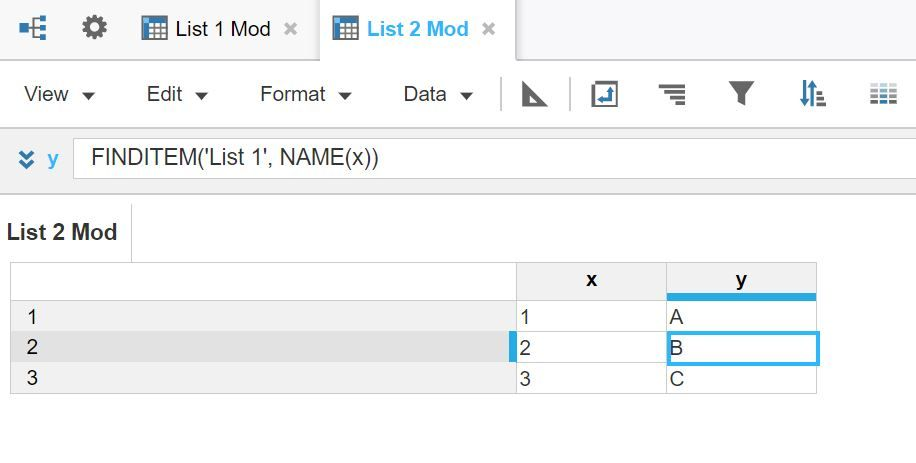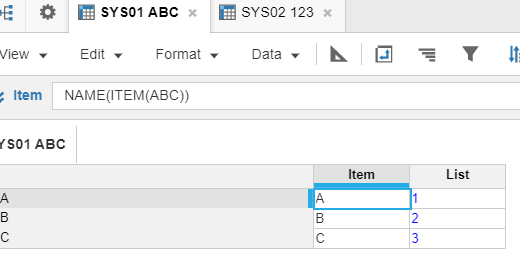# mapping of propertyHi,

I have created a list with members : A,B,C. This has a property with values 1,2,3 respectively which is also a list. Now in my list with 1,2,3 members, I need to map the values A,B,C (list) so that 1=A, 2=B, 3=C as shown in screenshot below :Is there a formula to automatically link the Property in List 123, to List  ABC highlighted in yellow?

Thanks,
Ana

Tagged:

•Hi @Ana22,

Is there any condition that you have to use only property.

Easy way is, if you assign code as 1,2,3 for List item A,B,C respectively.

Then using finditem() function you can easily get the result.Formula for x is item('Dimension List')As per Anaplan best practice, don't create properties instead create a properties module with corresponding line items which you have to set as properties.

Let me know if it helps.

•Hi, Thanks, but the 2 lists cant use the code column. They have different code convention. I just need it as property. If I create a module and add that as line item, what is the formula so I can link it?

Thanks,
Ana

•Hello Ana,

Could it happen that you will have one to many mapping?

1 = A, B

2 = C

or is there always a one to one mapping?

Regards,

Eije

•HI,

NO, it's one is to one. Or it could be as follows :

1=A

2=B

3=B

4=C

Thanks

Ana

•HI @Ana22,

Let's use the System modules to hold any attributes of a list as part of Anaplan Best Practice. You may have to use TEXTLIST functionality to achieve this result. Example below.Note: This would work only if there is one to one mapping between these 2 lists.

I hope it helps!